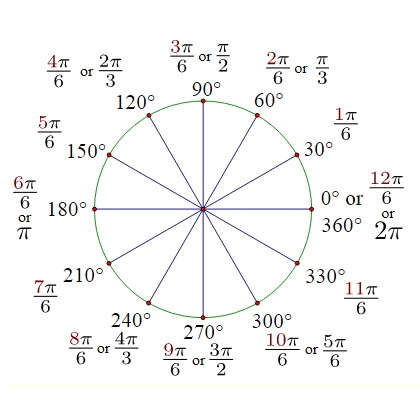The online conversion tool is used for a given angle to convert degree to radian and also vice-versa from radian to degree. Angles can be measured in units of either degrees or radians. The symbol for degree is ◦. Usually no symbol is used to denote radians.

A complete revolution is defined as 360◦ or 2π radians. π stands for the number 3.14159 . . . and you can work with this if you prefer. However in many calculations you will find that you need to work directly with multiples of π.### Degree Definition

Degrees: one degree (1°) is a rotation of 1/360 of a complete revolution about the vertex. We can think of this as going all the way around the circle. There are 360 degrees in one full rotation.

Radian: One Radian is the measure of a central angle θ that intercepts an arc s equal in length to the radius r of the circle. Since the radian is measured in terms one r on the arc of a circle and the complete circumference of the circle is 2πr then there are 2π radians in one full rotation. This gives us a way to convert between degrees and radians: